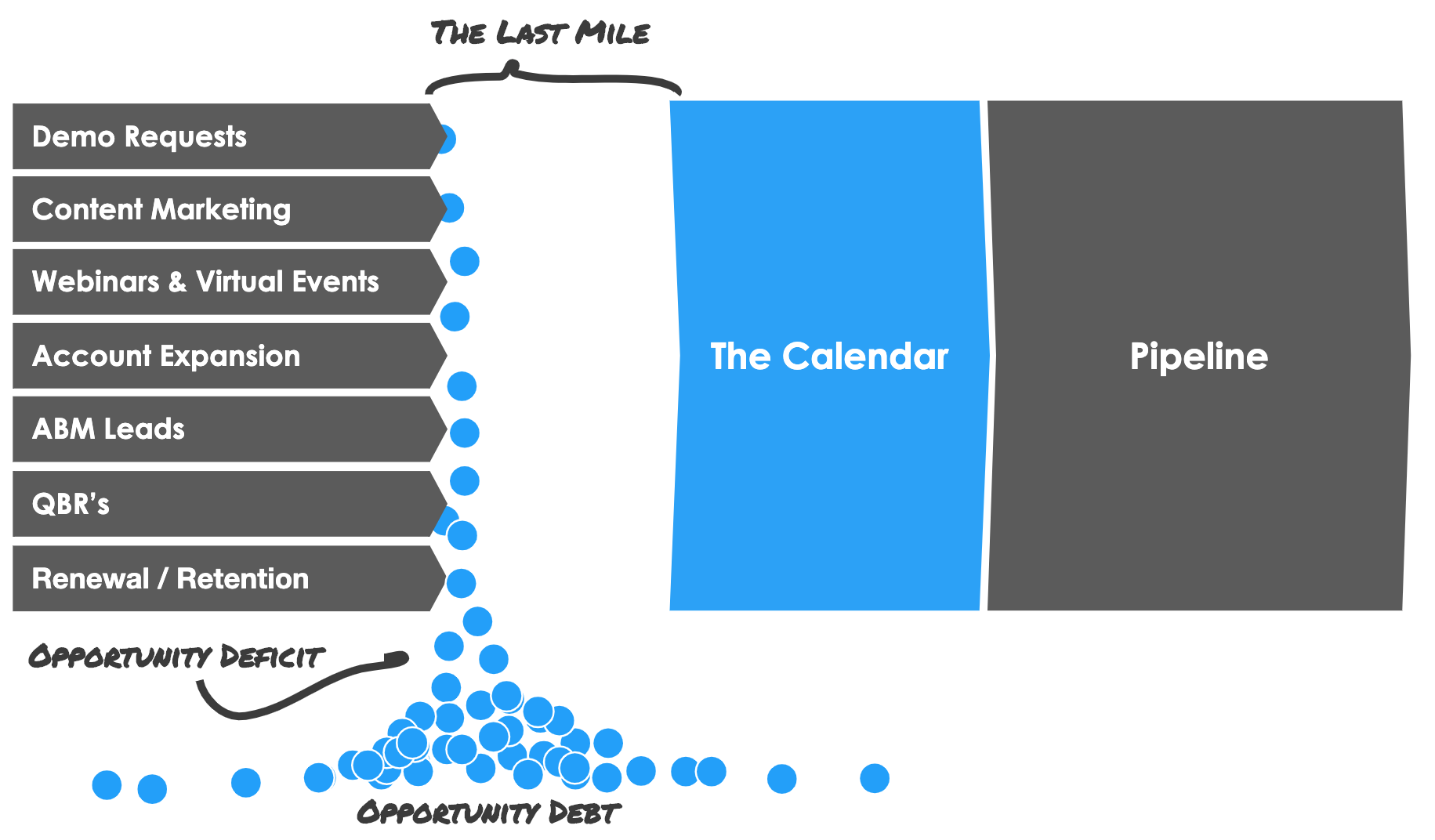Meeting Math is a surprisingly simple & accurate way to forecast future revenue. The larger the data-set for a given meeting type the more accurate the Meeting Math. Revenue leaders user meeting math as a forecasting tool that sits on-top of the pipeline, Meeting Math is also used when calculating Kronologic's ROI. Meeting Math is calculated on a per meeting type basis. There are only three required variables for basic meeting math.

Basic Meeting Math Formula

Average Contract Value
x Pipeline Win Rate
x Meeting to Pipeline Conversion

# Example

 Variables: Average Contract Value: \$10,000 Pipeline Win Rate: 20% Meeting to Pipeline Conversion: 50%

Do the Math

# Last Mile ProblemThe calculating the size of the last mile is a way to understand what is lost for a given channel, here we assume 100% conversion from lead to meeting to determine a starting point of what is left behind.  Calculating the size of a given last mile is easy.

Last Mile Formula